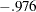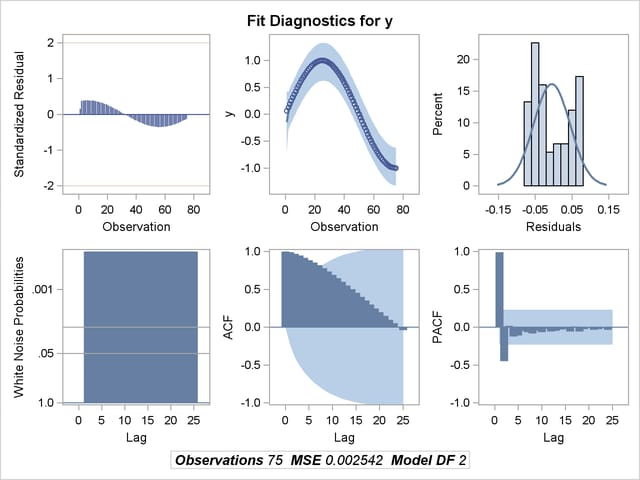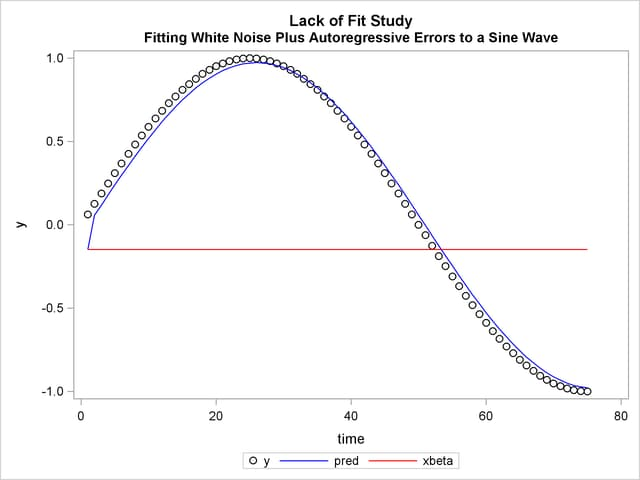The AUTOREG Procedure

## Example 8.3 Lack-of-Fit Study

Many time series exhibit high positive autocorrelation, having the smooth appearance of a random walk. This behavior can be explained by the partial adjustment and adaptive expectation hypotheses.

Short-term forecasting applications often use autoregressive models because these models absorb the behavior of this kind of data. In the case of a first-order AR process where the autoregressive parameter is exactly 1 (a random walk ), the best prediction of the future is the immediate past.

PROC AUTOREG can often greatly improve the fit of models, not only by adding additional parameters but also by capturing the random walk tendencies. Thus, PROC AUTOREG can be expected to provide good short-term forecast predictions.

However, good forecasts do not necessarily mean that your structural model contributes anything worthwhile to the fit. In the following example, random noise is fit to part of a sine wave. Notice that the structural model does not fit at all, but the autoregressive process does quite well and is very nearly a first difference (AR(1) =). The DATA step, PROC AUTOREG step, and PROC SGPLOT step follow:

```title1 'Lack of Fit Study';
title2 'Fitting White Noise Plus Autoregressive Errors to a Sine Wave';

data a;
pi=3.14159;
do time = 1 to 75;
if time > 75 then y = .;
else y = sin( pi * ( time / 50 ) );
x = ranuni( 1234567 );
output;
end;
run;
```
```proc autoreg data=a plots;
model y = x / nlag=1;
output out=b p=pred pm=xbeta;
run;
```
```proc sgplot data=b;
scatter y=y x=time / markerattrs=(color=black);
series y=pred x=time / lineattrs=(color=blue);
series y=xbeta x=time / lineattrs=(color=red);
run;
```

The printed output produced by PROC AUTOREG is shown in Output 8.3.1 and Output 8.3.2. Plots of observed and predicted values are shown in Output 8.3.3 and Output 8.3.4. Note: the plot Output 8.3.3 can be viewed in the Autoreg.Model.FitDiagnosticPlots category by selecting ViewResults.

Output 8.3.1 Results of OLS Analysis: No Autoregressive Model Fit
 Lack of Fit Study Fitting White Noise Plus Autoregressive Errors to a Sine Wave

The AUTOREG Procedure

Dependent Variable y

Ordinary Least Squares Estimates
SSE 34.8061005 DFE 73
MSE 0.47680 Root MSE 0.69050
SBC 163.898598 AIC 159.263622
MAE 0.59112447 AICC 159.430289
MAPE 117894.045 HQC 161.114317
Durbin-Watson 0.0057 Regress R-Square 0.0008
Total R-Square 0.0008

Parameter Estimates
Variable DF Estimate Standard Error t Value Approx
Pr > |t|
Intercept 1 0.2383 0.1584 1.50 0.1367
x 1 -0.0665 0.2771 -0.24 0.8109

Estimates of Autocorrelations
Lag Covariance Correlation -1 9 8 7 6 5 4 3 2 1 0 1 2 3 4 5 6 7 8 9 1
0 0.4641 1.000000 |                    |********************|
1 0.4531 0.976386 |                    |********************|

 Preliminary MSE 0.0217

Output 8.3.2 Regression Results with AR(1) Error Correction
Estimates of Autoregressive Parameters
Lag Coefficient Standard Error t Value
1 -0.976386 0.025460 -38.35

Yule-Walker Estimates
SSE 0.18304264 DFE 72
MSE 0.00254 Root MSE 0.05042
SBC -222.30643 AIC -229.2589
MAE 0.04551667 AICC -228.92087
MAPE 29145.3526 HQC -226.48285
Durbin-Watson 0.0942 Regress R-Square 0.0001
Total R-Square 0.9947

Parameter Estimates
Variable DF Estimate Standard Error t Value Approx
Pr > |t|
Intercept 1 -0.1473 0.1702 -0.87 0.3898
x 1 -0.001219 0.0141 -0.09 0.9315

Output 8.3.3 Diagnostics PlotsOutput 8.3.4 Plot of Autoregressive PredictionPrevious Page | Next Page | Top of Page